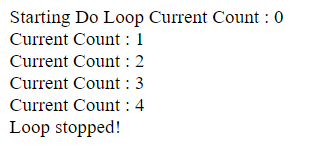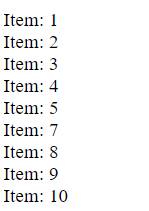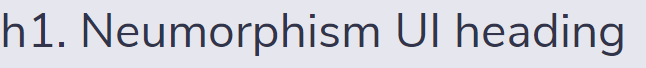# B4J Code Snippet[BANano]: Sharing the goodness

#### Mashiane

##### Expert
Longtime User
For Loops

The For Each Loop (the variable type on the loop should be defined)

B4X:
``````write("For Each Loop<br /> ")
Dim appNames() As String = Array("b4a", "b4i", "b4j", "b4r")
For Each appName As String In appNames
write(appName)
write("<br />")
Next
write ("Exiting from the loop!")``````

OutputThe Counter Loop

B4X:
``````write("Counter For Loop<br /> ")
'
Dim x As Int = 10
Dim y As Int
For y = 1 To x
write(\$"y: \${y}"\$)
write("<br />")
Next
write ("Exiting counter loop!")``````

OutputThe Step Down Loop

B4X:
``````Dim x As Int = 10
Dim y As Int
For y = 10 To 1 Step -1
write(\$"y: \${y}"\$)
write("<br />")
Next
write ("Exiting step counter loop!")``````•joulongleu

#### Mashiane

##### Expert
Longtime User
Exiting & Skipping Loops

This is based on a condition

1. Here we exit as soon as the counter is 5

B4X:
``````write("Starting Do Loop ")

Do Until (count = 10)
write(\$"Current Count : \${count}<br />"\$)
count = count + 1
'*****
If count = 5 Then Exit
Loop

write("Loop stopped!")``````

Output2. Here we skip and process the next loop line as soon as the item is 6

B4X:
``````Dim b As Int = 0
For b = 1 To 10
If b = 6 Then
Continue
End If
document.write(\$"Item: \${b}</br>"\$)
Next``````

outputLast edited:
•joulongleu

#### Mashiane

##### Expert
Longtime User
A helper class to add a BANanoElement in any parent element.

B4X:
``````Sub AddElement(parentID As String, tag As String, id As String) As BANanoElement
Dim el As BANanoElement = BANano.GetElement(\$"#\${parentID}"\$).Append(\$"<\${tag} id="\${id}"></\${tag}>"\$).Get(\$"#\${id}"\$)
Return el
End Sub``````

Usage

B4X:
``AddElement("body", "h1", "myh1").SetText("h1. Neumorphism UI heading")``

Meaning:

Find the #body element, append to it a h1 element, set the id of the newly created h1 element to myh1, then set the text to "h1. Neomorphism UI heading•joulongleu and Johan Hormaza

#### Mashiane

##### Expert
Longtime User
Check if your browser storage is marked for persistent storage?

B4X:
``````Sub BANano_Ready
Dim canPersist As Boolean = BANano.Await(BANano.Navigator.GetField("storage").RunMethod("persisted", Null))
Log(canPersist)

'pgIndex.Init
End Sub``````

•LJG

#### Mashiane

##### Expert
Longtime User
Request browser storage persistence

B4X:
``````'request persistence
Dim isPersisted As Boolean = BANano.Await(BANano.Navigator.GetField("storage").RunMethod("persist", Null))
Log(\$"Persisted storage granted: \${isPersisted}"\$)``````

Last edited:
•Rubsanpe and LJG

#### Mashiane

##### Expert
Longtime User
RunRecursive

B4X:
``````'run a recursive method
Sub RunRecursive(data As Map, path As String) As Object
Dim prevObj As BANanoObject = data
Dim items As List = BANano.Split(".", path)
Dim iTot As Int = items.Size
Dim iCnt As Int
'
Dim strprev As String = ""
Dim prtObj As BANanoObject
Dim litem As String = items.Get(iTot - 1)
'
For iCnt = 1 To iTot - 1
'get the previos path
strprev = items.Get(iCnt - 1)
'the parent object
prtObj = prevObj.GetField(strprev)
'this does not exist, return
If BANano.IsUndefined(prtObj) Then
Return Null
Else
prevObj = prtObj
End If
Next
Dim res As Object = prevObj.RunMethod(litem, Null).Result
If BANano.IsUndefined(res) Then
res = Null
End If
Return res
End Sub``````

Example code.. this executes a .RunMethod on the last path element delimited by .

Example Code

B4X:
``````Dim lat As Double = BANanoShared.RunRecursive(place, "geometry.location.lat")
Dim lng As Double = BANanoShared.RunRecursive(place, "geometry.location.lng")``````

Ta!

•joulongleu

#### Mashiane

##### Expert
Longtime User
B4X:
``````#if javascript
function dataURItoBlob(dataURI) {
// convert base64/URLEncoded data component to raw binary data held in a string
var byteString;
if (dataURI.split(',').indexOf('base64') >= 0)
byteString = atob(dataURI.split(','));
else
byteString = unescape(dataURI.split(','));

// separate out the mime component
var mimeString = dataURI.split(',').split(':').split(';');

// write the bytes of the string to a typed array
var ia = new Uint8Array(byteString.length);
for (var i = 0; i < byteString.length; i++) {
ia = byteString.charCodeAt(i);
}

return new Blob([ia], {type:mimeString});
}
#End If

'convert a dataURI to blob for form data
Sub dataURItoBlob(sDataURI As String) As BANanoObject
Dim out As BANanoObject = BANano.RunJavascriptMethod("dataURItoBlob", Array(sDataURI))
Return out
End Sub``````

#### Mashiane

##### Expert
Longtime User
Hex to RGB and back

B4X:
``````#if javascript
function rgbToHex(r, g, b) {
return "#" + ((1 << 24) + (r << 16) + (g << 8) + b).toString(16).slice(1);
}

function hexToRgb(hex) {
var result = /^#?([a-f\d]{2})([a-f\d]{2})([a-f\d]{2})\$/i.exec(hex);
if(result){
var r= parseInt(result, 16);
var g= parseInt(result, 16);
var b= parseInt(result, 16);
return r+","+g+","+b;//return 23,14,45 -> reformat if needed
}
return null;
}
#End If

'convert RGB to HEX
Sub RGBToHex(r As Int, g As Int, b As String) As String
Dim sout As String = BANano.RunJavascriptMethod("rgbToHex", Array(r, g, b))
Return sout
End Sub

'convert HEX to RGB
Sub HexToRGB(hexValue As String) As String
Dim sout As String = BANano.RunJavascriptMethod("hexToRgb", Array(hexValue))
Return sout
End Sub``````

#### Mashiane

##### Expert
Longtime User
'build URLSearchParams from a Map (used with BANanoFetch)

B4X:
``````Sub URLSearchParamsFromMap(params As Map) As BANanoObject
Dim obj As BANanoObject
obj.Initialize2("URLSearchParams", params)
Return obj
End Sub``````

build a URLQueryString from Map

B4X:
``````Sub URLQueryStringFromMap(params As Map) As String
Dim sb As StringBuilder
sb.Initialize
For Each k As String In params.Keys
Dim v As String = params.Get(k)
k = banano.EncodeURIComponent(k)
v = banano.EncodeURIComponent(v)
sb.Append(\$"\${k}=\${v}&"\$)
Next
Dim sout As String = sb.ToString
sout = BANanoShared.RemDelim(sout, "&")
Return sout
End Sub``````

•Johan Hormaza

#### Mashiane

##### Expert
Longtime User

B4X:
``````'show the browser notification
Sub ShowBrowserNotification(Title As String, Desc As String, Url As String, Icon As String, Vibrate As Boolean, Seconds As Int)        'ignoreDeadCode
Dim notif As BANanoObject = BANano.Window.GetField("Notification")
'check the permission
Dim permission As String = notif.GetField("permission").Result
If permission <> "granted" Then
'requestPermission
Dim resp As String = BANano.Await(notif.RunMethod("requestPermission", Null))
If resp <> "granted" Then Return
End If

Dim Options As Map = CreateMap()
Options.Put("icon", Icon)
Options.Put("body", Desc)
Options.Put("vibrate", Vibrate)
'
Dim n As BANanoObject
Dim showEvent As String = "notification_show"
Dim closeEvent As String = "notification_close"
Dim errorEvent As String = "notification_error"
Dim clickEvent As String = "notification_click"

If SubExists(EventHandler, showEvent) Then
End If
If SubExists(EventHandler, clickEvent) Then
End If
If SubExists(EventHandler, errorEvent) Then
End If
If SubExists(EventHandler, closeEvent) Then
End If
'close after
Dim cbClose As BANanoObject = BANano.callback(Me, "CloseNotification", Array(n))
Dim Sec As Int = Seconds * 1000
BANano.Window.SetTimeout(cbClose, Sec)
End Sub

n.RunMethod("close", Null)
End Sub``````

•Rubsanpe

#### Mashiane

##### Expert
Longtime User
Table Pagination Script in JavaScript

B4X:
``````"use strict";

var paginate = function paginate(items) {
var page = arguments.length > 1 && arguments !== undefined ? arguments : 1;
var perPage = arguments.length > 2 && arguments !== undefined ? arguments : 10;
var offset = perPage * (page - 1);
var totalPages = Math.ceil(items.length / perPage);
var paginatedItems = items.slice(offset, perPage * page);
return {
previousPage: page - 1 ? page - 1 : null,
nextPage: totalPages > page ? page + 1 : null,
total: items.length,
totalPages: totalPages,
items: paginatedItems
};
};``````